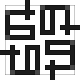Linda - 3 years ago 143
HTML Question

# Calling a javascript function from a fragment shader (HTML)

I am pretty new to webgl, and I've tried finding answers to my problem in old questions but I haven't been able to find any.

My problem is that I in my fragment shader need a function that generates a random number between -1 and 1. As I understand it I need to make a function myself, because HTML doesn't have a random number generator but Javascript does. I made this function in the head:

``````<script = "text/javascript">
function random() {
return Math.random()*2-1;    //random number between -1 and 1
}
</script>
``````

When I then try to call the function in the fragment shader, I am told that no overloaded function is found. But I can't really figure out how I am suppose to declare and call the function then. This is how I try to invoke it in the fragment shader:

`````` ...
for (float i = 0.0 ; i < no_of_samples ; i ++){

ndc.x = 2.0*((gl_FragCoord.x+half_pixelsize*random())/width-0.5);

...
``````transistor09